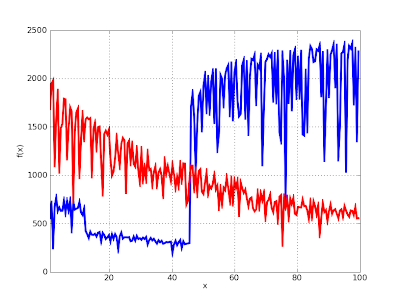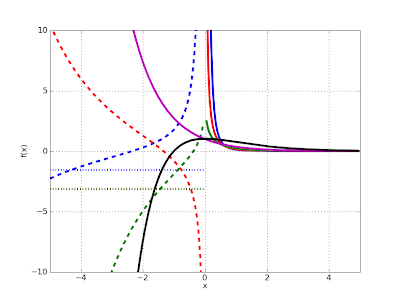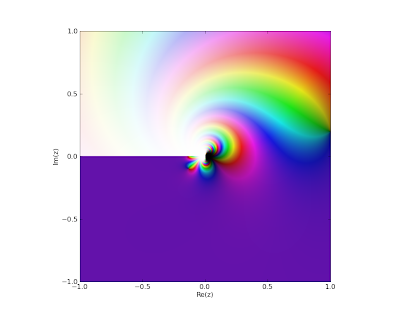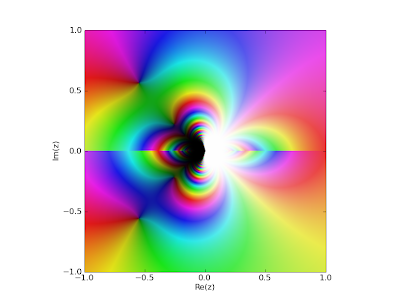# Improved incomplete gamma and exponential integrals; Clausen functions

July 10, 2009

The SVN trunk of mpmath now contains much improved implementations of the incomplete gamma function (gammainc()) as well as the exponential integrals (ei(), e1(), expint()). Although the code is not quite perfect yet, this was a rather tedious undertaking, so I’m probably going to work on something entirely different for a while and give these functions another iteration later.

The incomplete gamma function comes in three flavors: the lower incomplete gamma function, the upper incomplete gamma function, and the generalized (two-endpoints) incomplete gamma function. The generalized incomplete gamma function is defined aswhich reduces to the lower function when a = 0, and the upper version when b = +∞. A huge number of integrals occurring in pure and applied mathematics have this form (even Gaussian integrals, with a change of variables) so a solid incomplete gamma is quite important. It’s especially important to ensure both speed in correctness in asymptotic cases.

The lower incomplete gamma function is easiest to implement, because it’s essentially just a rescaled version of the hypergeometric 1F1 function and the 1F1 implementation already works well. Not much change was made here, so I’m going to write some about the other cases instead.

The exponential integrals are essentially the same as the upper incomplete gamma function and mostly share code. Remarks about the upper gamma function therefore also apply to the exponential integrals.

#### Upper gamma performance

The upper incomplete gamma function is hard because the two main series representations are an asymptotic series involving 2F0 that doesn’t always converge, and an 1F1 series that suffers badly from cancellation for even moderately large arguments. The problem is to decide when to use which series.

The 2F0 series is very fast when it converges, while the 1F1 series is quite slow (due to the need for extra precision) just below the point where 2F0 starts to converge. After some experimentation, I decided to change the implementation of 2F0. Instead of performing a heuristic, conservative test to determine whether the series will converge (sometimes claiming falsely that it won’t), it now always goes ahead to sum the series and raises an error only when it actually doesn’t converge.

Thus the asymptotic series will always be used when possible, and although this leads to a slight slowdown for smaller arguments, it avoids worst-case slowness. The most important use for the incomplete gamma function, I believe, is in asymptotics, so I think this is a correct priority.

As a result, you can now do this:

>>> from mpmath import *>>> mp.dps = 25; mp.pretty = True>>> gammainc(10, 100)4.083660630910611272288592e-26>>> gammainc(10, 10000000000000000)5.290402449901174752972486e-4342944819032375>>> gammainc(3+4j, 1000000+1000000j)(-1.257913707524362408877881e-434284 + 2.556691003883483531962095e-434284j)

The following graph compares old and new performance. The y axis shows the reciprocal time (higher is better) for computing gammainc(3.5, x) as x ranges between 0 and 100, at the standard precision of 15 digits. Red is the old implementation and blue is the new. The code also works with complex numbers, of course; replacing x with j*x gives a virtually identical graph (slightly scaled down due to the general overhead of complex arithmetic).It’s very visible where the asymptotic series kicks in, and the speed from then on is about 2000 evaluations per second which is relatively good. The new implementation is regrettably up to 3x slower than the old one for smaller x, although the slowdown is a bit misleading since the old version was broken and gave inaccurate results. The big dip in the blue graph at x = 10 is due to the automatic cancellation correction which the old code didn’t use.

The gap between the asymptotic and non-asymptotic cases could be closed by using specialized series code for the lower incomplete gamma function, or using the Legendre continued fraction for intermediate cases (this comes with some problems however, such as accurately estimating the rate of convergence, and the higher overhead for evaluating a continued fraction than a series). This will certainly be worth doing, but I’m not going to pursue those optimizations right now for reasons already stated.

Some good news is that the graph above shows worst-case behavior, where the generic code is used, due the parameter 3.5. I’ve also implemented fast special-purpose code for the case when the first parameter is a (reasonably small) integer. This also means that the exponential integrals E1(x), Ei(x) as well as En(x) for integer n can be evaluated efficiently.

Here is a speed comparison between the old and new implementations of the ei(x) function, again at standard precision. There is actually no change in algorithm here: the old implementation used a Taylor series for small arguments and an asymptotic series for large arguments. The difference is due to using only low-level code; this turned out to buy a factor 2 in the Taylor case and more than an order of magnitude (!) in the asymptotic case.The results are similar for the E1 function and with a complex argument. It is similar (only a bit slower) for gammainc(n,x) and expint(n,x) with a small integer value for n, although so far fast code is only implemented for real x in those cases.

#### Accurate generalized incomplete gamma function

The generalized incomplete gamma function can be written either as the difference of two upper gammas, or as the difference of two lower gammas. Which representation is better depends on the arguments. In general, one will work while the other will lead to total cancellation. gammainc is now clever enough to switch representations.

This uses a difference of lower gamma functions behind the scenes:

>>> gammainc(10000000, 3) - gammainc(10000000, 2)   # Bad0.0>>> gammainc(10000000, 2, 3)   # Good1.755146243738946045873491e+4771204

This uses a difference of upper gamma functions behind the scenes:

>>> gammainc(2, 0, 100000001) - gammainc(2, 0, 100000000)   # Bad0.0>>> gammainc(2, 100000000, 100000001)   # Good4.078258353474186729184421e-43429441

#### Some demo plots

Here are two plots of the upper gamma functions and exponential integrals (for various values of the first parameter). A lot of time went into getting the correct branch cuts in the low-level code (and writing tests for them), so please appreciate the view of the imaginary parts.

T1 = lambda x: gammainc(-2,x)T2 = lambda x: gammainc(-1,x)T3 = lambda x: gammainc(0,x)T4 = lambda x: gammainc(1,x)T5 = lambda x: gammainc(2,x)plot([T1,T2,T3,T4,T5],[-5,5],[-10,10])T1 = lambda x: expint(-2,x)T2 = lambda x: expint(-1,x)T3 = lambda x: expint(0,x)T4 = lambda x: expint(1,x)T5 = lambda x: expint(2,x)plot([T1,T2,T3,T4,T5],[-5,5],[-10,10])And a complex plot of gammainc(3+4j, 1/z):A plot of gammainc(1/z, -1/z, 1/z); a rather nonsensical function (but that is besides the point):#### Clausen functions

Unrelated to the gamma functions, I’ve also implemented Clausen functions:

These functions are just polylogarithms in disguise, but convenient as standalone functions. With them one can evaluate certain divergent Fourier series for example:

>>> clsin(-2, 3)-0.01781786725924798006896962>>> nsum(lambda k: k**2 * sin(3*k), [1,inf])-0.01781786725924798006896962

They also work for complex arguments (and are related to zeta functions):

>>> clsin(2+3j, 1+2j)(1.352229437254898401329125 + 1.401881614736751520048876j)>>> clcos(2+3j, pi)(-1.042010539574581174661637 - 0.2070574989958949174656102j)>>> altzeta(2+3j)(1.042010539574581174661637 + 0.2070574989958949174656102j)>>> chop(clcos(zetazero(2), pi/2))0.0